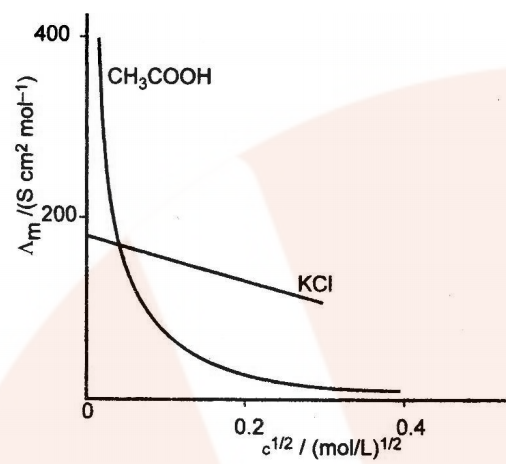## Conductance (G)

It is the reciprocal of resistance and may be defined as the ease with which the electric current flows through a conductor.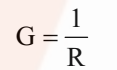SI unit is Siemen (S).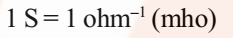### The electronic conductance depends on:

• the nature and structure of the metal
• the number of valence electrons per atom
• temperature (it decreases with the increase of temperature)

## Conductivity (k)

It is the reciprocal of resistivity (k).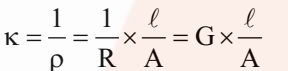Now if l = 1 cm and A = 1 cm2, then k = G.
Hence, the conductivity of an electrolytic solution may be defined as the conductance of a solution of 1 cm length with the area of cross-section equal to 1 cm2.

### The conductivity of electrolytic (ionic) solutions depends on:

• the nature of the electrolyte added
• size of the ions produced and their solvation
• the nature of the solvent and its viscosity
• the concentration of the electrolyte
• temperature (it increases with the increase of temperature).

### Factoring Affecting Electrolyte Conductance

Electrolyte: An electrolyte is a substance that dissociates in solution to produce ions and hence conducts electricity in the dissolved or molten state.

The conductance of electricity by ions present in the solutions is called electrolytic or ionic conductance. The following factors govern the flow of electricity through a solution of electrolyte.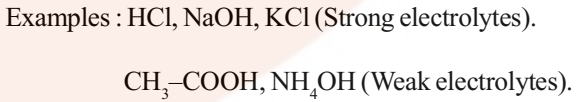The conductance of electricity by ions present in the solutions is called electrolytic or ionic conductance. The following factors govern the flow of electricity through a solution of electrolyte.

• Nature of electrolyte or interionic attractions: Lesser the solute-solute interactions, greater will be the freedom of movement of ions and higher will be the conductance.
• Solvation of Ions: Larger the magnitude of solute-solvent interactions, greater is the extent of solvation and lower will be the electrical conductance.
• The nature of the solvent and its viscosity: Larger the solvent-solvent interactions, larger will be viscosity and more will be the resistance offered by the solvent to flow of ions and hence lesser will be the electrical conductance.
• Temperature: As the temperature of the electrolytic solution rises solute-solvent, solvent-solvent interactions decreases, this results in the increase of electrolytic conductance.

## Measurement of Conductance

As we know,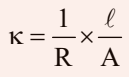The value of k could be known, if we measure l, A and R. The value of the resistance of the solution R between two parallel electrodes is determined by using Wheatstone’s bridge method (Fig.)

It consists of two fixed resistance R3 and R4, a variable resistance R1 and the conductivity cell having the unknown resistance R2. The bridge is balanced when no current passes through the detector. Under these conditions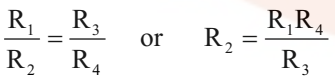## Molar conductivity (ʌm)

It may be defined as the conducting power of all the ions produced by dissolving one mole of an electrolyte placed between two large electrodes at one centimetre apart.
Mathematically,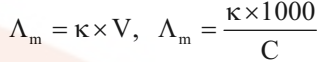where V is the volume of solution in cm3 containing 1 mole of electrolyte and C is the molar concentration.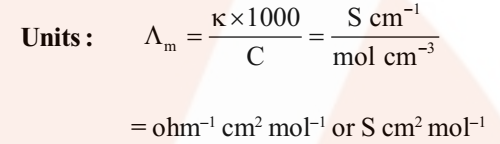## Equivalent Conductivity (ʌeq)

It is conducting power of one equivalent of electrolyte placed between two large electrodes at one centimeter apart.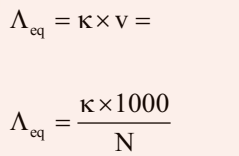Where v is the volume of solution in cm3 containing 1 equivalent of electrolyte and N is normality.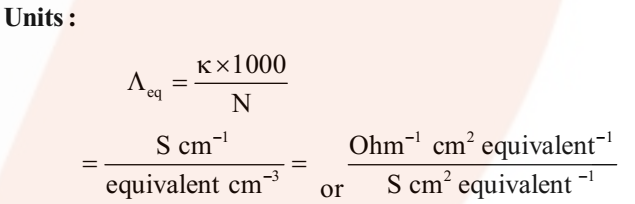### Variation of Conductivity and Molar Conductivity with Dilution

Conductivity decreases with the decrease in concentration, this is because the number of ions per unit volume that carry the current in the solution decreases on dilution.
Molar conductivity (ʌm = k x V) increases with the decrease in concentration. This is because the total volume V of the solution containing one mole of electrolyte also increases.

It has been found that the decrease in on dilution of a solution is more than compensated by increases in its volume.

### Graphic representation of the variation of ʌm vs c1/2